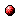SSCWeb + FEEDBACKSSC Users Guide - Table of Contents

APPENDIX C: Description of Selected Coordinate Systems Used in SSC Programs

1. GEI: Geocentric Equatorial Inertial system. This system has X-axis pointing from the Earth toward the first point of Aries (the position of the Sun at the vernal equinox). This direction is the intersection of the Earth's equatorial plane and the ecliptic plane and thus the X-axis lies in both planes. The Z-axis is parallel to the rotation axis of the Earth, and y completes the right-handed orthogonal set (Y = Z * X). Geocentric Inertial (GCI) and Earth-Centered Inertial (ECI) are the same as GEI.

2. GEO: Geographic coordinate system. This system is defined so that its X-axis is in the Earth's equatorial plane but is fixed with the rotation of the Earth so that it passes through the Greenwich meridian (0 longitude). Its Z-axis is parallel to the rotation axis of the Earth, and its Y-axis completes a right handed orthogonal set (Y = Z * X).

3. GM: Geomagnetic coordinate system. Z-axis points to the Geomagnetic north pole (in Greenland). The positive X-axis points towards the great circle encompassing the North and South Geomagetic poles and lies in the geomagnetic equatorial plane in the segment that is in the western hemisphere. (The South GM pole is the antipode of the North GM pole.) Earth-centered Dipole is invoked. Y completes the triad.

4. GSE: Geocentric Solar Ecliptic system. This has its X-axis pointing from the Earth toward the Sun and its Y-axis is chosen to be in the ecliptic plane pointing towards dusk (thus opposing planetary motion). Its Z-axis is parallel to the ecliptic pole. Relative to an inertial system this system has a yearly rotation.

5. GSM: Geocentric Solar Magnetospheric system. This has its X-axis from the Earth to the Sun. The Y-axis is defined to be perpendicular to the Earth's magnetic dipole so that the X-Z plane contains the dipole axis. The positive Z-axis is chosen to be in the same sense as the northern magnetic pole. The difference between the GSM and GSE systems is simply a rotation about the X-axis.

6. SM: Solar Magnetic coordinates. In this system, the Z-axis is chosen parallel to the north magnetic pole and the Y-axis perpendicular to the Earth-Sun line towards dusk. The difference between this system and the GSM system is a rotation about the Y-axis. The amount of rotation is simply the dipole tilt angle. We note that in this system the X-axis does not point directly at the Sun. As with the GSM system, the SM system rotates with both a yearly and daily period with respect to inertial coordinates.

7. Invariant Latitude: For any point in space one can trace a B-field line to the Earth surface, assuming it is a centered dipole field. The GM latitude of this foot point is labelled as the Invariant Latitude along the entire field line. The dipole L-value is closely related to this invariant latitude; L=1/(Cos(Lat))^2, and physically connotes the distance (in Earth radii) of the "top of the field line" from Earth center.

8. J2000: Geocentric Equatorial Inertial for epoch J2000.0 (GEI2000), also known as Mean Equator and Mean Equinox of J2000.0 (Julian date 2451545.0 TT (Terrestrial Time), or 2000 January 1 noon TT, or 2000 January 1 11:59:27.816 TAI or 2000 January 1 11:58:55.816 UTC.) This system has X-axis aligned with the mean equinox for epoch J2000; Z-axis is parallel to the rotation axis of the Earth, and Y completes the right-handed orthogonal set.

Reference:"Geophysical Coordinate Transformations", C.T. Russell, Cosmic Electrodynamics, Vol. 2, pp. 184 - 196, 1971SSC Users Guide - Table of Contents